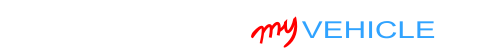## Interpreting Your Compression Test Results

If your engine compression test results show one or more cylinders with low compression values, the next step is to find out they're causing a problem.

To find out if the lower compression value is causing an engine performance problem, you need to find out if it's lower than 15% of the highest compression value you got.

You can do this (figuring out the 15%) in one of two ways: You can calculate this 15% difference with pen and paper or you can use my low compression calculator. You can find the low compression calculator here: Online Low Engine Compression Calculator (at: easyautodiagnostics.com).

If you want to manually calculate the 15% difference, here's what you'll need to do:

1. STEP 1: Multiply the highest compression value by 0.15 (this is the decimal value of 15%).
2. STEP 2: Round the result to the nearest one (for example: 25.6 would become 26).
3. STEP 3: Subtract the result (the number that was rounded) from the highest compression value.
4. ANSWER: The result of this subtraction is the lowest possible compression value any cylinder can have.

Now, let me give you a more specific example: Let's say that I got the following compression readings:

Cylinder Pressure
#1 165 PSI
#2   95 PSI
#3 155 PSI
#4 175 PSI

My next step is to do the following calculation:

1. STEP 1:  175 x 0.15 = 26.25.
2. STEP 2:  26.25 = 26 (rounded to nearest one).
3. STEP 3:  175 - 26 = 149.
4. ANSWER:  149 PSI. Any cylinder with this compression (or lower) value will misfire.

Since cylinder #2 is only producing 95 PSI, I can now conclude that it's 'dead' and causing a misfire.

To find out if the lowest compression value you got from your engine compression test is within a good range, you'll need to do the same calculation. Of course, you'll need to use the highest compression value you got and not the one in the example.

Once you've found the 'dead' cylinder, the next step is to find out what's causing the low compression value. For this step, go to: TEST 2: ‘Wet’ Engine Compression Test.

## TEST 2: ‘Wet’ Engine Compression Test

Once you've found the cylinder or cylinders with no or low engine compression, the next step is to do a wet compression test on them.

A wet compression test simply involves adding about 2 tablespoons of oil the cylinder and checking its compression again.

Let me explain, low or no engine compression in a cylinder is usually do to a problem with its piston rings or its cylinder head valves.

By adding two tablespoons of engine oil (to the low or no compression cylinder), we'll be able to find out if the problem is due to bad piston rings or bad cylinder head valve valves.

If the problem is due to bad piston rings, the engine oil will help to compress the air in the cylinder and the compression value on your test gauge will go up.

But if the problem is in the cylinder head valve, the oil will not be able to help them seal the air being compressed and the reading on your test gauge will remain the same as the reading from TEST 1.

OK, this is what you need to do:

1. 1

Add a small amount of engine oil to the cylinder that reported low compression or no compression in the ‘Dry’ compression test.

The amount should be about 1 to 2 tablespoons of oil.

2. 2

Install the compression tester onto the cylinder.

Do not use any type of tool to tightened the compression tester. Hand tight is fine.

3. 3

When all is set up, have your helper crank the engine.

4. 4

You'll get one of two results:

1.) The compression value will go up (from the one you recorded before).

2.) The compression value will stay the same.

CASE 1: The compression value, of the cylinder you added oil to, went up. This tells you that the problem causing the low or no compression value is bad piston rings on this specific cylinder.

CASE 2: The compression value, of the cylinder you added oil to, DID NOT go up. This test results tells you that the problem causing the low or no compression reading in TEST 1 is due to bad cylinder head valves.If this info saved the day, buy me a beer!